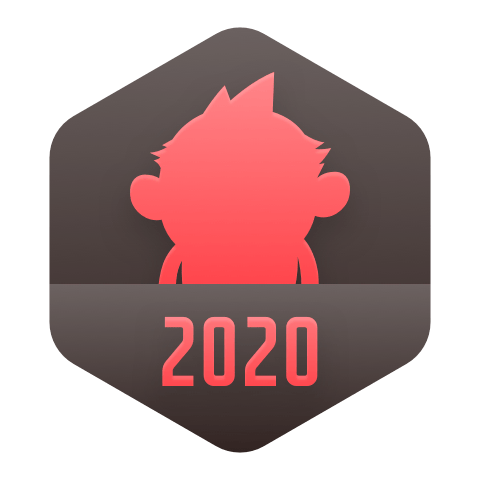### 如何把字符转换为字符串？

C/C++ > C++ 语言 [问题点数：20分，结帖人ariesjzj]ariesjzjC++ - 将长整型数转换为字符串

Java - 数据类型之间的转换：如何将字符串转换为基本数据类型？如何将基本数据类型转换为字符串？

【java】把字符串转换为字符数组

package test922; import java.util.Arrays; public class StringToCharTest { public static void main(String[] args) { String line = "abcdefg"... char[] chs=line.toCharArray();...

C语言 16行代码实现字符串中指定字符串替换

Java中字符数组与字符串转换方法

c++字符串与c字符串

Python has different variable types. Integer and string types are the most used types. And the conversation between them is required most of the time. In this post, we will look at howto convert strin...

C#判断字符串是否数字字符串

java把字符串转换成日期格式

public static void main(String[] args) throws Exception{ Date date = new SimpleDateFormat("yyyy-MM-dd").parse("2005-06-09"); String now = new SimpleDateFormat("yyyy年MM月dd日").format(date);...

java新手：字符串数组、字符数组和字符串之间的转换

（1）字符串和字符数组的转化 // 字符串转化成字符数组 String str = &quot;abcdefg&quot;; char[] ch = str.toCharArray(); //输出a System.out.println(ch); //字符数组转化成字符串 ...

【Python】字符串转换为ASCII码

Python将字符串转换为列表

We can convert a string to ... 我们可以使用split（）函数将字符串转换为Python中的列表。 Python String split() function syntax is: Python字符串split（）函数语法： str.split(sep=None, maxsplit=-1)...

Java中数字转换为字符串字符串转换为字符

java数字和字符串相互转化

7-4 字符串字母大小写转换 （15 分）

java中字符数组与字符串之间互相转换的方法

public static void main...字符数组 转换字符串 //(1)直接在构造String时转换 char[] array = new char[] {'a','b','c','d','e','f','g'}; String str = new String(array); System.out.println(str...

//数组里的字符串转换成数字的方法 ...//数组中数字转换字符串[1, 2, 3].map(String) //结果['1', '2', '3'] 其它：['1', '2', '3'].map(returnInt); // [1, 2, 3] // 意料之中的结果['1', '...

js字符串转换为对象格式

a="[1,2,3]" newa=a.replace('"','').replace(']','').replace('[','') print([ int(i) for i in newa.split(',')]) ''' [1, 2, 3] ''' ...newa=a.replace('\'[','').replace(']\'','') ...

Beanshell中字符串拆分及字符串转换为整型

c++将整数转换为字符串字符串转化数字

Java 实现把字符串转换成整数

C语言 整数与字符串的相互转换

C语言 整数与字符串的相互转换 一、简述  C语言中整数与字符串的相互... itoa (表示 integer to alphanumeric)是整型数转换字符串的一个函数。  windows环境下，在&lt;stdlib.h&gt;头文件中有 c...

JAVA把字符串转换成整数

Java语言将JSON字符串转换为Map

c++将字符转换字符串